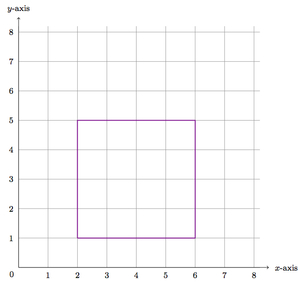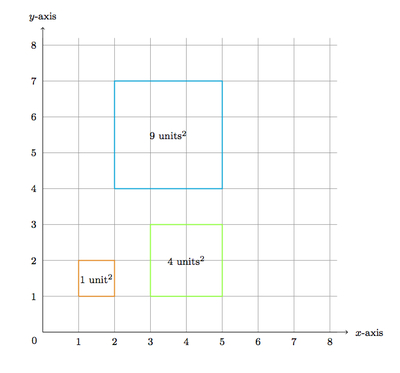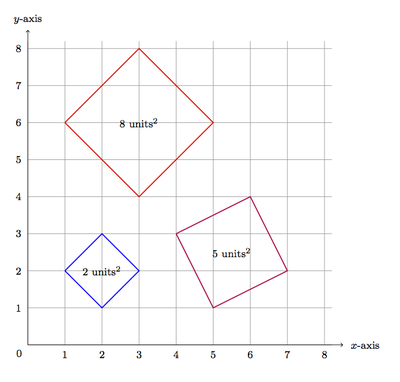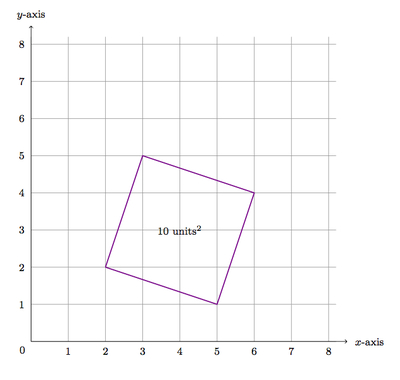# Squares on a coordinate grid

Alignments to Content Standards: G-GPE.B.7

## Task

In the picture below a square is outlined whose vertices lie on the coordinate grid points:The area of this particular square is 16 square units. For each whole number $n$ between 1 and 10, find a square with vertices on the coordinate grid whose area is $n$ square units or show that there is no such square.

## IM Commentary

The purpose of this task is to use the Pythagorean Theorem and knowledge about quadrilaterals in order to construct squares of different sizes on a coordinate grid. In addition to the Pythagorean Theorem, students will need to show that the shapes they have constructed are squares. This can be done by using the Pythagorean Theorem a second time to check that, in addition to having four congruent sides, the quadrilaterals also have congruent diagonals. Alternatively, as is done in the solution, they can check that the lines containing intersecting sides of the square are perpendicular. Addressing all of these issues will require patience and skill, making the task ideally suited for group work. In addition, the task builds upon many different strands of knowledge and so it is recommended for use with experienced students.

This task leads to many interesting questions:

• In general, for which numbers $n$ can we find a square on the grid whose area is $n$ square units? For which $n$ is there no such square? This line of reasoning leads to some very interesting questions in number theory.
• What other polygons can we find with vertices on the coordinate grid? Can we find an equilateral triangle? A regular hexagon? A parallelogram with specified angles? These are all challenging and interesting questions.

The idea for this task came from an Albuquerque Math Teachers' Circle meeting on October 1, 2013.

## Solution

For $n = 1, 2, 4, 5, 8, 9, 10$ there are squares of area $n$ units$^2$. The easiest squares to visualize are those having areas of $1, 4,$ and $9$ units$^2$:The next diagram shows examples of squares whose areas are 2, 5, and 8 units$^2$:The last diagram shows a square of area 10 units$^2$:We will first explain why the oblique squares have the desired areas and then work on showing that they are squares. The different cases are similar so we focus on the diagram above with the square of area 10 units$^2$. We first choose a pair of adjacent vertices (2,2) and (3,5). According to the Pythagorean Theorem the length of this side is $$\sqrt{(2-3)^2 + (2-5)^2} = \sqrt{10} \text{ units}.$$ We can check similarly that all of the other sides have length $\sqrt{10}$ units and so, assuming the shape is a square, its area is 10 units$^2$ as desired. A similar argument will work for each of the other figures.

For the angles, the side joining (2,2) to (5,1) has slope $$\frac{2-1}{2-5} = -\frac{1}{3}.$$ The side joining (5,1) to (6,4) has slope $$\frac{1-4}{5-6} = 3.$$ These slopes have a product of $-1$ and so the two lines containing these segments are perpendicular. Similar calculations show that the other angles in this quadrilateral are right angles and so it is a square.

A second way to show that these are squares is to use the fact that a square is a quadrilateral which is both a rectangle and a rhombus and then use properties of rectangles and rhombi. For example, a square is a rhombus whose diagonals are congruent. Working with the last quadrilateral again (which we have shown to be a rhombus by calculating its side lengths), the diagonal joining (2,2) to (6,4) has length $$\sqrt{(6-2)^2+(4-2)^2} = \sqrt{20} \text{ units}.$$ The other diagonal joining (3,5) and (5,1) has length $$\sqrt{(3-5)^2 + (5-1)^2} = \sqrt{20} \text{ units}.$$ The two diagonals of the rhombus are congruent and so it is a square. Similar calculations can be made for any of the other squares. Alternatively, a square is a rectangle whose diagonals are perpendicular. We have seen how to show that the quadrilateral is a rectangle. Its diagonals have slopes of $-2$ and $\frac{1}{2}$. Since the product of these slopes is $-1$, the diagonals are perpendicular, and the quadrilateral is a square.

Lastly, we need to explain why there are no squares of area 3, 6, and 7 units$^{2}$ whose vertices lie at grid points. Suppose $(a_1,b_1)$ and $(a_2,b_2)$ are vertices of a side of a square. Then the length of that side is $$\sqrt{(a_2-a_1)^2 + (b_2-b_1)^2} \text{ units}^2$$ and so the area of the square can be written as a sum of squares: $$(a_2-a_1)^2 + (b_2-b_1)^2 \text{ units}^2.$$ If the vertices of the square lie on grid points then $a_2 - a_1$ and $b_2 - b_1$ are integers. We can then check that 3, 6, and 7 cannot be written as sums of squares of integers and so there are no squares with these areas whose vertices lie on grid points.